## Quadratic Equation: Optimization without Calculus

In this section, I will show an illustrative example on simple optimization problem (to maximize some objective) and its solution using quadratic function.

### Example

Suppose a farmer has a large piece of land and he wants to make a rectangular fence for his animals but he has no money to buy more wood for the fence. Therefore, the total length of the fence is fixed to be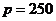meters. What should the width and length of the rectangle such that the area is maximized?

### Solution:

Suppose the width is denoted by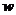and the length is denoted by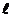. The perimeter of the rectangle is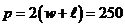and the area of rectangle is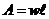. From the perimeter equation, we can write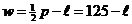and inputting this into the area of rectangle, we obtain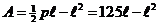.

This is a quadratic function in the form of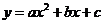where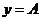,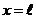with parameters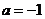,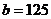and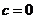.We will use the characteristic of quadratic function to solve this optimization problem. Since the quadratic parameter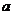is negative, we have sad parabola with maximum extreme point at coordinate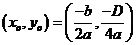where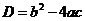is the discriminant. Inputting the values of parameters into the extreme point coordinate, we have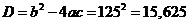The area of rectangle is maximized at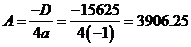square meter.

The length of the rectangle is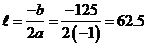meter

The width is computed as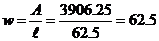meter

Thus, the area of the fenced land is maximized if the boundary is a square with side of 62.5 meter.

Note: Knowing that square shape yield largest area when the perimeter is bounded by the amount of wood he has (meters), now the farmer asks if he makes the region as circle, will he get larger area or smaller area. The perimeter of circle is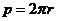and area of a circle is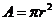. Inputting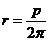into the equation of area of circle yields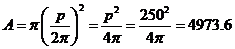square meter, which is actually larger than a square with the same perimeter.

Notice that we do not use any derivative or calculus to solve the optimization problems above.

Go to the next section to find out more resources on quadratic function, equation and formula

Preferable reference for this tutorial is